# Help understanding this extrapolation technique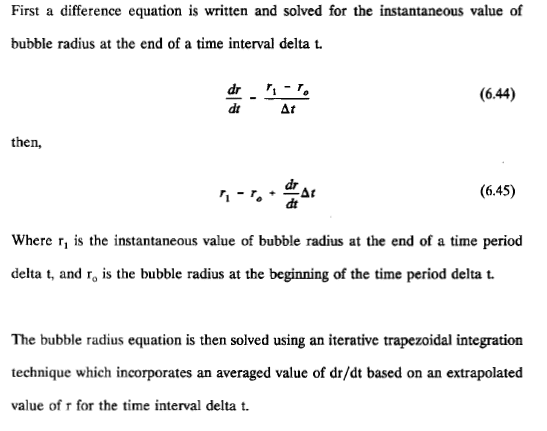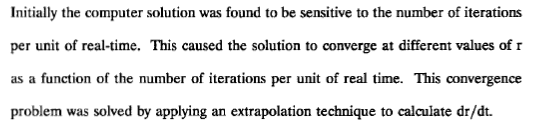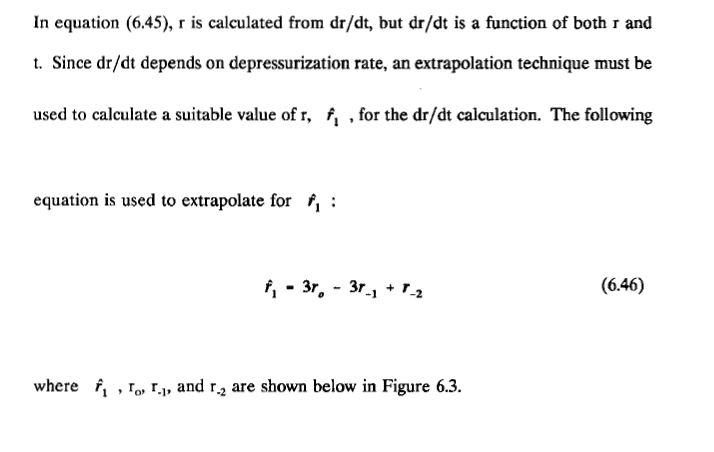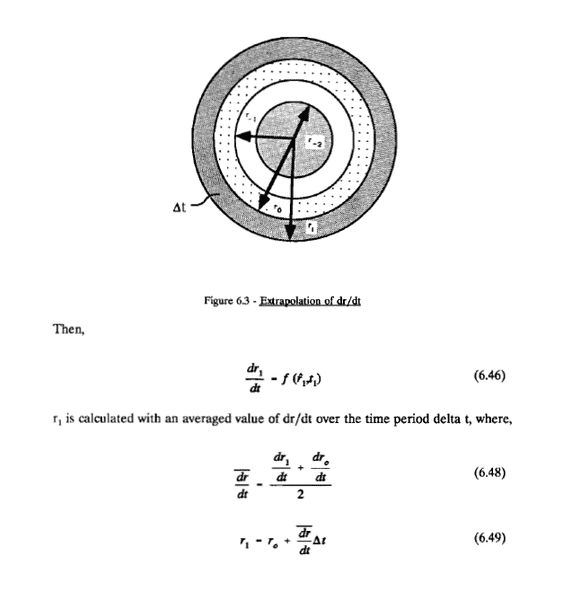Trying to understand what is going on here, and how this technique removes the dependence of sample rate from the solution.

RUber
Homework Helper
If you Taylor expand out the function in time, you get for time step h:
##r(t+h) = r(t) + h r'(t) + \frac{h^2}{2} r''(t) + \frac{h^3}{3!} r'''(t)...\\
r(t - h) = r(t) - h r'(t) + \frac{h^2}{2} r''(t) -\frac{h^3}{3!} r'''(t)... \\
r(t-2h) = r(t) - 2h r'(t) + \frac{4h^2}{2} r''(t) -\frac{8h^3}{3!} r'''(t)... ##
##3r(t) - 3r(t-h) + r(t-2h) = r(t) +h r'(t) + \frac{h^2}{2} r''(t) -\frac{5h^3}{6} r'''(t)... ##
So you can see this scheme is accurate to ##\mathcal{O}(h^3). ##

The benefit of this method is that it looks like an semi-implicit method, which should become stable regardless the sample rate. The accuracy will still be dependent on sample rate, but the stability is much improved compared to forward difference methods.

If you Taylor expand out the function in time, you get for time step h:
##r(t+h) = r(t) + h r'(t) + \frac{h^2}{2} r''(t) + \frac{h^3}{3!} r'''(t)...\\
r(t - h) = r(t) - h r'(t) + \frac{h^2}{2} r''(t) -\frac{h^3}{3!} r'''(t)... \\
r(t-2h) = r(t) - 2h r'(t) + \frac{4h^2}{2} r''(t) -\frac{8h^3}{3!} r'''(t)... ##
##3r(t) - 3r(t-h) + r(t-2h) = r(t) +h r'(t) + \frac{h^2}{2} r''(t) -\frac{5h^3}{6} r'''(t)... ##
So you can see this scheme is accurate to ##\mathcal{O}(h^3). ##

The benefit of this method is that it looks like an semi-implicit method, which should become stable regardless the sample rate. The accuracy will still be dependent on sample rate, but the stability is much improved compared to forward difference methods.

Would it be possible to plot the radius change over time in matlab? How would I go about doing that?

RUber
Homework Helper
In the attachment, the equation marked (6.46) says
##\frac{dr_1}{dt} = f(\hat r _1, t_1).##
Do you know that function? If not, you can approximate the derivatives simply, but you lose some accuracy.

Here is a rough code for the approximation:
-------------------------------------------------------------

function R = bubble(r0,r1,r2, N, dt)
% the output of will be a Nx2 matrix with the first column as the radius
% and the second column the first derivative in time. Inputs are the radius
% at three known times (r1,r2,r3), the number of timesteps (N),
% the step size (dt), and the function f.

%initialize with initial conditions
rnow = r2;
rnowless1 = r1;
rnowless2 = r0;

R = zeros(N+3,1);
R(1) = r0;
R(2) = r1;
R(3) = r2;
%timestep routine
for step = 1:N
% guess at rnext.
rhat = 3*rnow -3*rnowless1 + rnowless2; %eq 6.46 from text
rbardot = (rhat-rnowless1)/(2*dt); %this is the same as [(rhat-rnow)/dt + (rnow-rnowless1)/dt]/2
rnext = rnow + rbardot*dt;
R(step+3) = rnext; %save radius data.
% reset for new step
rnowless2 = rnowless1;
rnowless1 = rnow;
rnow = rnext;
end

plot(0:dt:(N+2)*dt,R);
end

•lostinphysics44
In the attachment, the equation marked (6.46) says
##\frac{dr_1}{dt} = f(\hat r _1, t_1).##
Do you know that function? If not, you can approximate the derivatives simply, but you lose some accuracy.

The function is the overall bubble size as it relates to a variety of factors (pressure, time, viscosity, tissue tension, etc) I am trying to understand how to plot the radius over time as pressure changes. From what you explained it seems that at each dt there is a pressure (which I can set based on my ascent or descent rate) that I can plug in to the equation and get a new radius of bubble. Am I supposed to calculate the output of that equation using the extrapolation technique you just explained?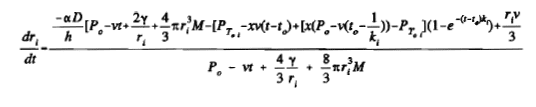So my steps in matlab as far as I can tell would be:
1. Set dt timestep
2. set pressure rate (PT in the equation, Po is the initial pressure)
3. plug that into the 6.46 equation and solve to get a radius value
4. use that radius value as the new initial radius and repeat until the time or pressure ends.
The paper I am reading says that dr/dt is a first order non linear differential equation solved using the trapezoid rule. Is that separate from what you described with your previous response?

RUber
Homework Helper
That seems to work, remember that you are using the approximation ##\hat r_1 ## to approximate the derivative, so plug that estimate (based on prior r data) into the formula for dr/dt.
Once you have that estimate for dr/dt, you apply the averaging and approximation in 6.48 and 6.49.

Assuming you know the previous time data ##r_{i-1},r_{i-2},r_{i-3}##:
1. Set dt timestep.
2. Set pressure rate.
3. Calculate approximation ##\hat r_i## based on previous r information.
4. Use formula with pressure and ##\hat r_i## or ## r_{i-1}## to get ##\frac{dr_i}{dt}## and ##\frac{dr_{i-1}}{dt}##
5. Use equation 6.48 to get average rate of change for the timestep.
6. Use equation 6.49 to approximate ##r_i##.

That seems to work, remember that you are using the approximation ##\hat r_1 ## to approximate the derivative, so plug that estimate (based on prior r data) into the formula for dr/dt.
Once you have that estimate for dr/dt, you apply the averaging and approximation in 6.48 and 6.49.

Assuming you know the previous time data ##r_{i-1},r_{i-2},r_{i-3}##:
1. Set dt timestep.
2. Set pressure rate.
3. Calculate approximation ##\hat r_i## based on previous r information.
4. Use formula with pressure and ##\hat r_i## or ## r_{i-1}## to get ##\frac{dr_i}{dt}## and ##\frac{dr_{i-1}}{dt}##
5. Use equation 6.48 to get average rate of change for the timestep.
6. Use equation 6.49 to approximate ##r_i##.

In order for this to work I have to assume a starting bubble radius yes? This is probably not the right forum for this, but think if i took a shot at a matlab script you could look it over for me?

RUber
Homework Helper
Based on the example, it looks like you would want to have the radius at the first 3 times. You can surely assume that r = 0 or r = r0 for times prior to start.
Yes, I can look at a script for you.

Based on the example, it looks like you would want to have the radius at the first 3 times. You can surely assume that r = 0 or r = r0 for times prior to start.
Yes, I can look at a script for you.

Quick question: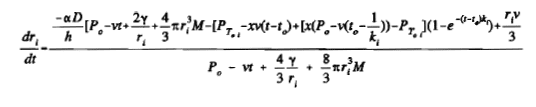in this equation, how do I solve it to get the dr_i/dt and dr_i-1/dt so that I can plug them into the averaging formula? I know everything and all the constants but what is the matlab way to solve this?

RUber
Homework Helper
If you know ##r_{i-1}##, you just plug it in. There is nothing to solve.
For the current (i) timestep, you don't know ##r_i##, so you use the ##\hat r_i## that you already computed.